# 【算法】蓝桥杯dfs深度优先搜索之凑算式总结

## 导航

→《【算法】蓝桥杯dfs深度优先搜索之图连通总结》

## 前言

2015年（第六届）蓝桥杯B组省赛

DFS/爆破 3（9分）、5（15分）、7（21分） 45分

（加法乘法）

6（17分） 17分

（饮料换购）

8（13分） 13分

DP 10（31分） 31分
2016年（第七届）蓝桥杯B组省赛

DFS/爆破

3（9分）、5（13分）、

6（15分）、7（19分）、

8（21分）

77分
DP 9（23分） 23分

2017年（第八届）蓝桥杯B组省赛

DFS/爆破 2（11分）、4（17分） 28分

DP 6（9分）、8（21分） 30分

2018年（第九届）蓝桥杯B组省赛

DFS/爆破 9（23分） 23分
DP 4（17分）、10（25分） 42分?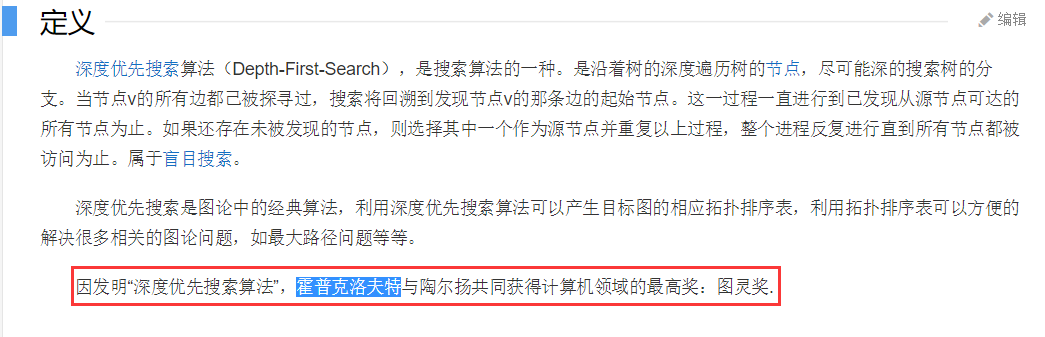?## 正文

### 【第一道题】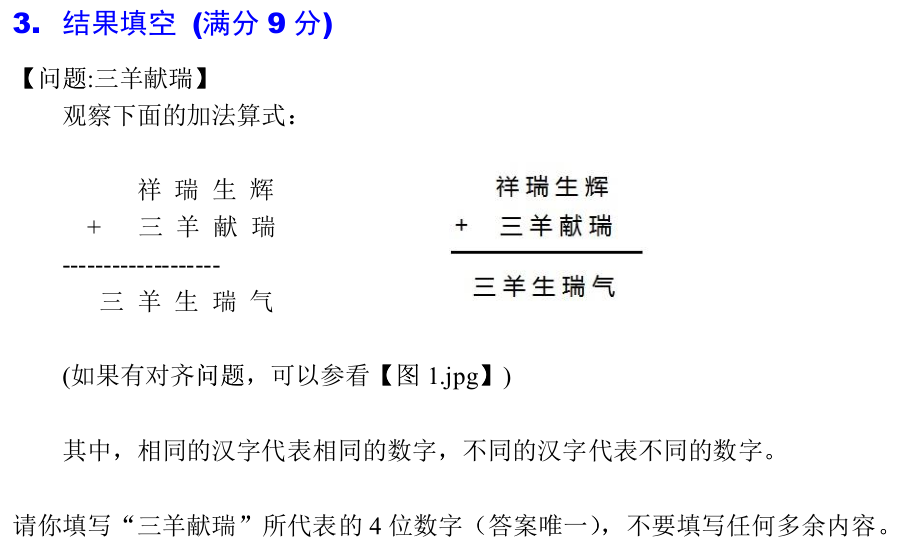?【分析】

a对应有下标index：0至7

``public static void dfs(int index)````````if(index == 8) {
int sum = (a*1000+a*100+a*10+a) + (a*1000+a*100+a*10+a);
if(sum == a*10000+a*1000+a*100+a*10+a)
System.out.println(a+""+a+""+a+""+a);
return;
}````````````for(int i=0; i<=9; i++) {
if(visited[i] == 0) {// 代表没被访问过，可以访问
visited[i] = 1; // 访问 i, 做标记
a[index] = i; // 往数组里放数
dfs(index+1); // 枚举下一种情况
visited[i] = 0; // 回溯, 清除标记
}
}````````````for(int i=0; i<=9; i++) {
if(index == 0 && i == 0) {// 祥字不可能为0，否则就无法进位了
continue;
}
if(index == 4 && i != 1) {// 三字必为1，因为十进制进位只能进1
continue;
}
if(visited[i] == 0) {// 代表没被访问过，可以访问
visited[i] = 1; // 访问 i, 做标记
a[index] = i;
dfs(index+1);
visited[i] = 0; // 回溯, 清除标记
}
}``````【完整代码】

``` 1 /**
2  *
3  *   祥瑞生辉
4  * + 三羊献瑞
5  * ----------
6  * 三羊生瑞气
7  *
8  *  抽象为如下：
9  *
10  *     aaaa
11  *  +  aaaa
12  *-------------------
13  * aaaaa
14  *
15  */
16 public class Main {
17     static int[] a = new int; // 总共有8个不同的字，代表8个不同的数
18     static int[] visited = new int[] {0,0,0,0,0,0,0,0,0,0}; // 10个0, 标记0-9十个数是否被访问过
19     public static void dfs(int index) {
20         // index为8，越界，代表8个数找够了，同时也是递归结束条件
21         if(index == 8) {
22             int sum = (a*1000+a*100+a*10+a) + (a*1000+a*100+a*10+a);
23             if(sum == a*10000+a*1000+a*100+a*10+a)
24                 System.out.println(a+""+a+""+a+""+a);
25             return;
26         } else {// 不够8个数，0-9十个数，深搜
27             for(int i=0; i<=9; i++) {
28                 if(index == 0 && i == 0) {// 祥字不可能为0，否则就无法进位了
29                     continue;
30                 }
31                 if(index == 4 && i != 1) {// 三字必为1，因为十进制进位只能进1
32                     continue;
33                 }
34                 if(visited[i] == 0) {// 代表没被访问过，可以访问
35                     visited[i] = 1; // 访问 i, 做标记
36                     a[index] = i;
37                     dfs(index+1);
38                     visited[i] = 0; // 回溯, 清除标记
39                 }
40             }
41         }
42
43     }
44
45     public static void main(String[] args) {
46         dfs(0);
47     }
48
49 }```### 【第二道题】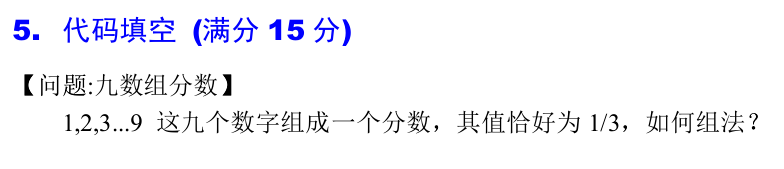?【分析】

``public static void dfs(int[] a, int index) ````````if(index == 9) {
int m = a*1000+a*100+a*10+a;
int n = a*10000+a*1000+a*100+a*10+a;
if(m*3 == n)
System.out.println(m+" "+n);
return;
}````````````for(int i=index; i<a.length; i++){
int t=a[index]; a[index]=a[i]; a[i]=t; // 交换a[index]和a[i]
dfs(a,index+1);
t=a[index]; a[index]=a[i]; a[i]=t; // 再次交换a[index]和a[i]
}``````【完整代码】

``` 1 public class Main {
2
3     public static void dfs(int[] a, int index) {
4                 // 结束条件
5         if(index == 9) {
6             int m = a*1000+a*100+a*10+a;
7             int n = a*10000+a*1000+a*100+a*10+a;
8             if(m*3 == n)
9                 System.out.println(m+" "+n);
10             return;
11         }
12
13         for(int i=index; i<a.length; i++){
14             int t=a[index]; a[index]=a[i]; a[i]=t; // 交换a[index]和a[i]
15             dfs(a,index+1);
16             t=a[index]; a[index]=a[i]; a[i]=t; // 再次交换a[index]和a[i]
17         }
18     }
19     public static void main(String[] args) {
20             int[] a = new int[]{1,2,3,4,5,6,7,8,9};
21         dfs(a,0);
22     }
23
24 }```### 【第三道题】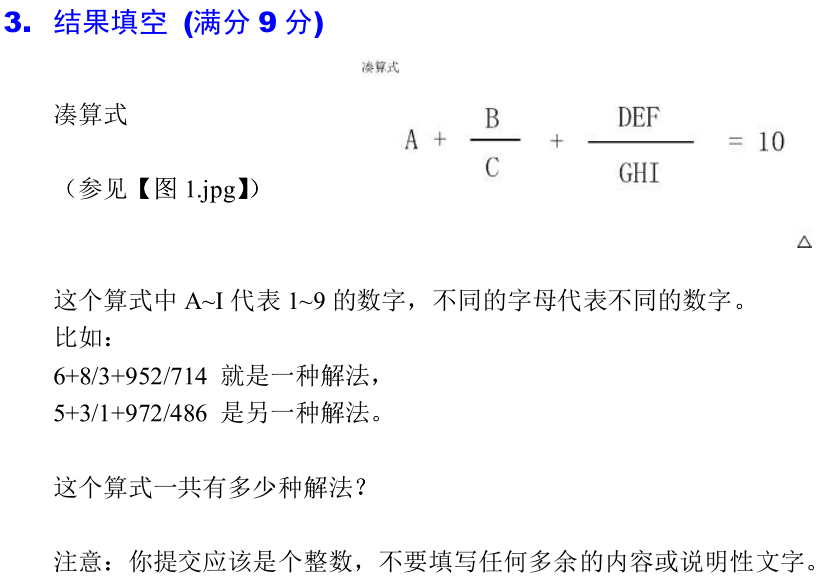?index还是int的，取值范围为0至8

dfs方法定义如下：

``public static void dfs(double[] a,int index)````````if(index == 9) {
if(a+a/a+(a*100+a*10+a)/(a*100+a*10+a) == 10)
count++;
return;
}````````````for(int i=index; i<a.length; i++) {
double t = a[index]; a[index]=a[i]; a[i] = t;
dfs(a,index+1);
t = a[index]; a[index]=a[i]; a[i] = t;
}``````【完整代码】

``` 1 public class 凑算式交换法dfs {
2
3     public static int count = 0;
4     public static void dfs(double[] a,int index) {
5         // 结束条件
6         if(index == 9) {
7             if(a+a/a+(a*100+a*10+a)/(a*100+a*10+a) == 10)
8                 count++;
9             return;
10         } else {
11             for(int i=index; i<a.length; i++) {
12                 double t = a[index]; a[index]=a[i]; a[i] = t;
13                 dfs(a,index+1);
14                 t = a[index]; a[index]=a[i]; a[i] = t;
15             }
16         }
17     }
18
19     public static void main(String[] args) {
20         double[] a = new double[] {1.00,2.00,3.00,4.00,5.00,6.00,7.00,8.00,9.00};
21         dfs(a,0);
22         System.out.println(count);
23     }
24
25 }```
``` 1 public class 凑算式交换法dfs {
2
3     public static int count = 0;
4     public static void dfs(double[] a,int index) {
5         // 结束条件
6         if(index == 9) {
7             if(a+a/a+(a*100+a*10+a)/(a*100+a*10+a) == 10)
8                 count++;
9             return;
10         } else {
11             for(int i=index; i<a.length; i++) {
12                 double t = a[index]; a[index]=a[i]; a[i] = t;
13                 dfs(a,index+1);
14                 t = a[index]; a[index]=a[i]; a[i] = t;
15             }
16         }
17     }
18
19     public static void main(String[] args) {
20         double[] a = new double[] {1.00,2.00,3.00,4.00,5.00,6.00,7.00,8.00,9.00};
21         dfs(a,0);
22         System.out.println(count);
23     }
24
25 }```【标记法完整代码】

``` 1 public class 凑算式标记dfs {
2     public static double[] a = new double;
3     public static int[] visited = new int[] {0,0,0,0,0,0,0,0,0}; // 9个0
4     public static int count = 0;
5     public static void dfs(int index) {
6         // 结束条件
7         if(index == 9) {
8             if(a+a/a+(a*100+a*10+a)/(a*100+a*10+a) == 10)
9                 count++;
10             return;
11         } else {
12             for(int i=1; i<=9; i++) {
13                 if(visited[i-1] == 0) { // 因为i从1开始，所以需要 -1
14                     visited[i-1] = 1; // 标记
15                     a[index] = (double)i;
16                     dfs(index+1);
17                     visited[i-1] = 0; // 清除标记
18                 }
19             }
20         }
21     }
22
23     public static void main(String[] args) {
24         dfs(0);
25         System.out.println(count);
26     }
27
28 }```Java语言int型数组初始化时会自动赋值为0，但是我为了直观表达，所以选择了显式初始化。

### 【第四道题】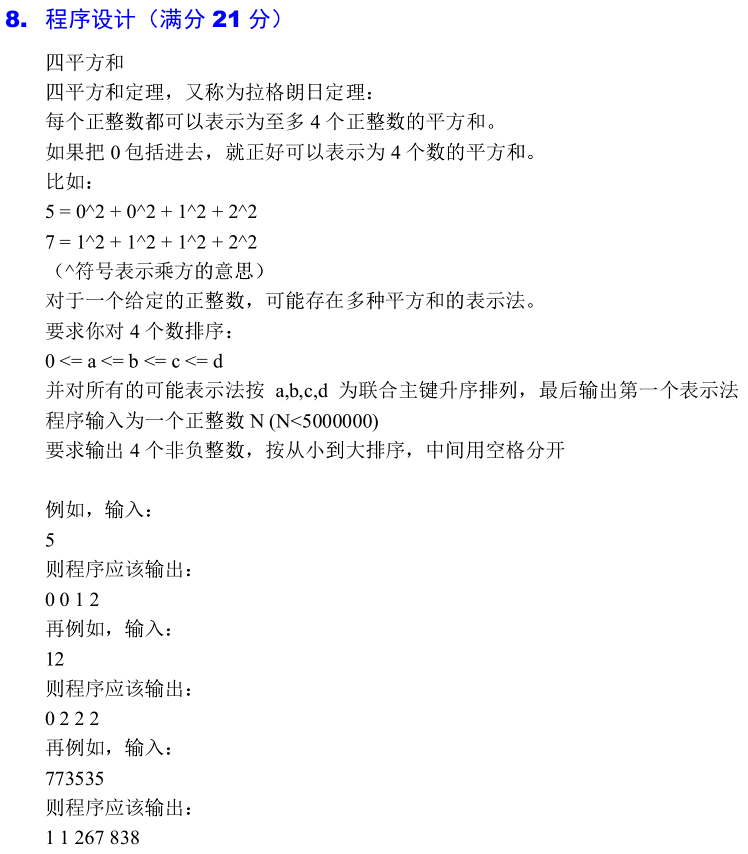?``public static void dfs(int index, int num)````````if(index == 4) {
if(num == (int)(Math.pow(a, 2)+Math.pow(a, 2)
+Math.pow(a, 2)+Math.pow(a, 2))) {
System.out.println(a+" "+a+" "+a+" "+a);
}
return;
}````````````for(int i=0; i<=(int)Math.sqrt(num); i++) {
a[index] = i;
dfs(index+1,num);
}``````【完整代码】

``` 1 import java.util.Scanner;
2
3 public class 四平方和dfs {
4
5     public static int[] a = new int;
6
7     public static void dfs(int index, int num) {
8     // 结束条件
9         if(index == 4) {
10             if(num == (int)(Math.pow(a, 2)+Math.pow(a, 2)
11                                 +Math.pow(a, 2)+Math.pow(a, 2))) {
12                 System.out.println(a+" "+a+" "+a+" "+a);
13             }
14             return;
15         }
16         // 搜索
17         for(int i=0; i<=(int)Math.sqrt(num); i++) {
18             a[index] = i;
19             dfs(index+1,num);
20         }
21     }
22
23     public static void main(String[] args) {
24         Scanner sc = new Scanner(System.in);
25         int num = sc.nextInt();
26         dfs(0,num);
27 }```【等等，这道题还没完】

``````5
0 0 1 2
0 0 2 1
0 1 0 2
0 1 2 0
0 2 0 1
0 2 1 0
1 0 0 2
1 0 2 0
1 2 0 0
2 0 0 1
2 0 1 0````````````System.out.println(a+" "+a+" "+a+" "+a);
System.exit(0); // 加上这句，打印完直接结束程序``````【再等最后一下，真的】

【CSDN】有梦就不怕痛《蓝桥杯 四平方和》

Java版代码如下（运行飞一般的流畅）：

``` 1 import java.util.Scanner;
2
3 public class 四平方和爆破 {
4
5     public static void main(String[] args) {
6         int n, a, b, c, d;
7         Scanner sc = new Scanner(System.in);
8         n = sc.nextInt();
9         for (a = 0; a < 3000; ++a) {// 3000² = 900万 > 500万
10             for (b = a; b < 3000; ++b) {
11                 for (c = b; c < 3000; ++c) {
12                     d = (int)Math.sqrt(n - a * a - b * b - c * c);
13                     if (n == a * a + b * b + c * c + d * d) {
14                         if (c > d) {
15                             int temp = d;
16                             d = c;
17                             c = temp;
18                         }
19                         System.out.println(a+" "+b+" "+c+" "+d);
20                         return; // 找到之后就return，让所有循环结束
21                     }
22                 }
23             }
24         }
25     }
26 }```### 【第五道题】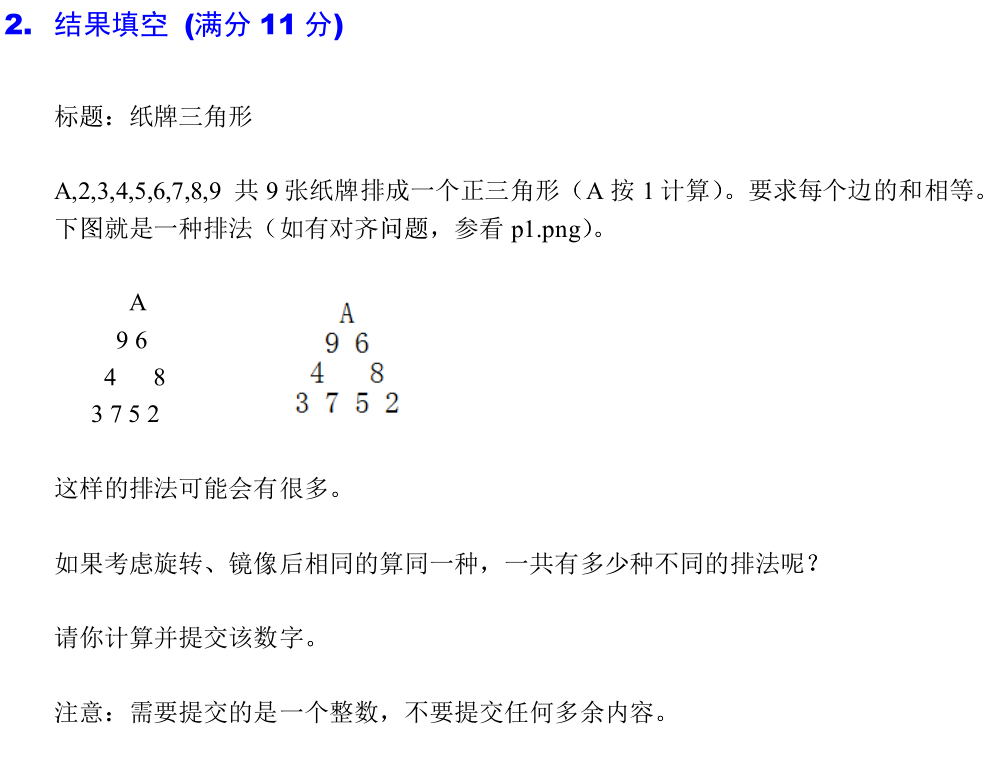?【完整代码】

``` 1 public class 纸牌三角形dfs {
2
3     public static int[] a = new int;
4     public static int[] visited = new int[] {0,0,0,0,0,0,0,0,0};
5     public static int count = 0;
6     public static void dfs(int index) {
7         // 结束条件
8         if(index == 9) {
9             if(a+a+a+a == a+a+a+a &&
10                 a+a+a+a == a+a+a+a) {
11                 count++;
12             }
13             return;
14         }
15         // 搜索
16         for(int i=1; i<=9; i++) {
17             if(visited[i-1] == 0) { // i从1开始，所以要i-1
18                 visited[i-1] = 1;
19                 a[index] = i;
20                 dfs(index+1);
21                 visited[i-1] = 0;
22             }
23         }
24     }
25
26     public static void main(String[] args) {
27         dfs(0);
28         System.out.println(count/6);
29     }
30
31 }```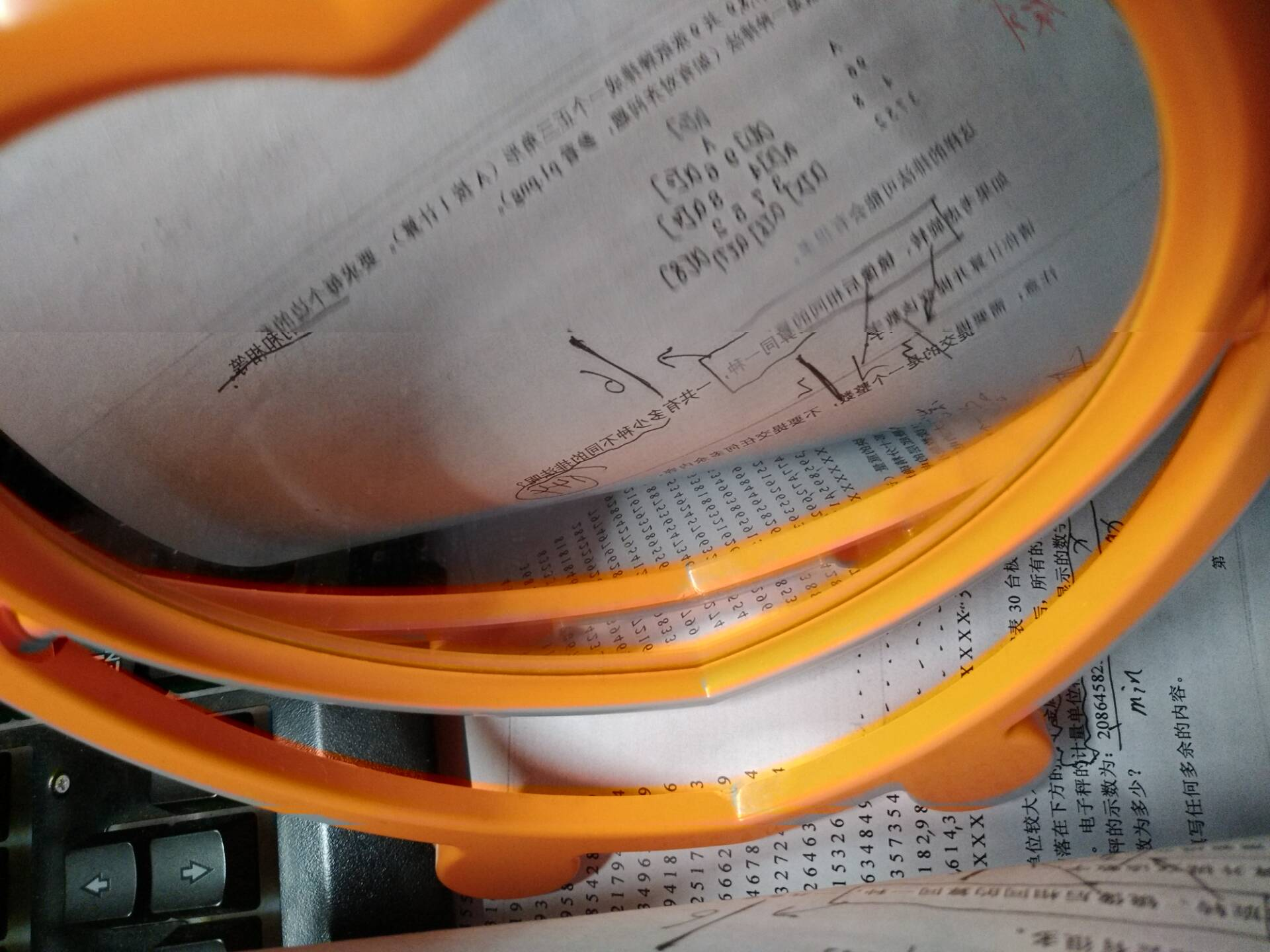?《【算法】蓝桥杯dfs深度优先搜索之排列组合总结》

【参考文章】

1. 【CSDN】梅森上校《JAVA版本：DFS算法题解两个例子（走迷宫和求排列组合数）》
2. 【CSDN】winycg《2015蓝桥杯 三羊献瑞（回溯法dfs）》
3. 【CSDN】有梦就不怕痛《蓝桥杯 四平方和》
4. 还有一些CSDN、博客园、简书.......由于浏览篇幅太多，所以一些未列在其中，表示抱歉

【算法】蓝桥杯dfs深度优先搜索之凑算式总结

(0)
(0)